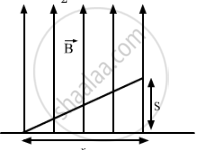Advertisement Remove all ads

# An Electron is Projected Horizontally with a Kinetic Energy of 10 Kev. a Magnetic Field of Strength 1.0 × 10−7 T Exists in the Vertically Upward Direction. (A) Will - Physics

Answer in Brief

An electron is projected horizontally with a kinetic energy of 10 keV. A magnetic field of strength 1.0 × 10−7 T exists in the vertically upward direction.
(a) Will the electron deflect towards the right or left of its motion?
(b) Calculate the sideways deflection of the electron while travelling through 1 m. Make appropriate approximations.

Advertisement Remove all ads

#### Solution

Given:
The kinetic energy of the electron projected in the horizontal direction, K.E = 10 keV = 1.6 × 10−15 J
Magnetic field, B = 1 × 10−7 T
The direction of magnetic field is vertically upward.
(a) The direction can be found by the right-hand screw rule. So, the electron will be deflected towards left.
(b) Kinetic energy,
K.E  = 1/2mv^2

v = sqrt((K.Exx 2)/m)
Magnetic force,
vecF =  q vecv xx vecB ......(i)
and F = ma
Therefore, equation (i) will be
a=(qvecvxx vecB)/m
Applying equation of motion
s = ut + 1/2 at^2t= Time taken to cross the magnetic field
As there is no force acting on the electron in the horizontal direction, the velocity of the electron remain constant in this direction.
So, the time taken to cross a distance of 1m in the horizontal direction in the magnetic field,
t = (d)/(v_"horizontal")

= (1)/sqrt((2xxkxxE)/m

= (qvB)/(4xxK.E)

= 1/4 (1.6xx10^-(19) xx1xx10^-7xx sqrt(2 xx K. E)/m)/(10xx 10^-16)

= 0.148 xx 10^-3 m
= 0.01482  cm
s = 0.0148 = 1.5 × 10−2 cm

Concept: Motion in Combined Electric and Magnetic Fields - Cyclotron
Is there an error in this question or solution?
Advertisement Remove all ads

#### APPEARS IN

HC Verma Class 11, Class 12 Concepts of Physics Vol. 2
Chapter 12 Magnetic Field
Q 2 | Page 230
Advertisement Remove all ads

#### Video TutorialsVIEW ALL 

Advertisement Remove all ads
Share
Notifications

View all notifications

Forgot password?